Unpivoting data is a common task when working with spreadsheets, as it involves transforming data from a wide format to a long format, making it easier to analyze and visualize. With the introduction of new functions in Excel, the process of unpivoting data has become even more straightforward and efficient.

This article aims to demonstrate how you can utilize Excel functions to convert pivoted sales data into a long format. By following the step-by-step instructions provided, you will gain valuable insights on the process of transforming your data using Excel's functions exclusively.

## Sales Data in Pivot Format

Consider an example where we have an input grid that allows salespeople to record the sales amount of each product on specific dates. The table is designed in a compact form, with dedicated columns assigned to different products, enhancing both display and ease of data entry. However, while this table format simplifies basic calculations such as determining the total number of products, it poses limitations when attempting to generate comprehensive reports that require detailed insights.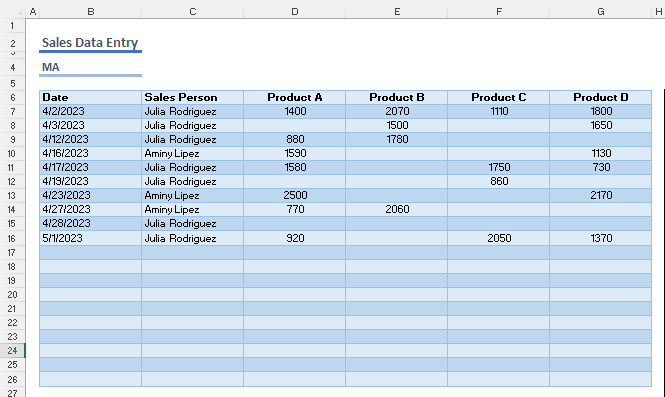The essential component of reports is a data set that hasn't been pivoted. In such a data set, each asset is assigned its own column, replete with categories and values. This configuration allows you to dictate the placement and representation of items from any column, whether it be in rows, columns, or as consolidated data.

## Unpivoting Data with Functions

Unpivoting is the process of reshaping data, converting it from columns to rows, such that the column headings form one row, and the rows of data beneath the columns merge into a single, distinct row. This restructuring of values is made simple with the help of a new array function, TOCOL. This function is specifically designed to render a specified array into a single row.As each row and column comprises multiple values, it's necessary to multiply them to achieve a matching quantity of values. This can be accomplished in several ways.

The most common approach involves generating a repeating sequence of numbers for each intersecting label, and then using a lookup function to fetch the labels. Take for instance, a sample data set with 10 rows and 4 columns - in this case, each row label should recur 4 times. Therefore, a sequence resembling this would be required: 1, 1, 1, 1, 2, 2, 2, 2, …., 10, 10, 10, 10.

We can populate this sequence easily by using the following formula:

`=QUOTIENT(SEQUENCE(10*4,,0),4)+1`

The SEQUENCE function generates forty numbers (10 rows * 4 columns) starting from 0. We can employ  the QUOTIENT function to divide these numbers to column count (4) to obtain the integer portion. As the quotient of the first four numbers equals to zero (0), we sum these numbers by 1.

You can use this repeating sequence of numbers with a traditional function like INDEX. However, in this article, we’ll use a new function called CHOOSEROWS.

`=CHOOSEROWS(array,row_num1,[row_num2],…)`

The CHOOSEROWS function returns the specified rows of a given array. Because we can generate the row numbers with the above function, the CHOOSEROWS function can return the rows we need.

`=CHOOSEROWS(<array>,<row number>)`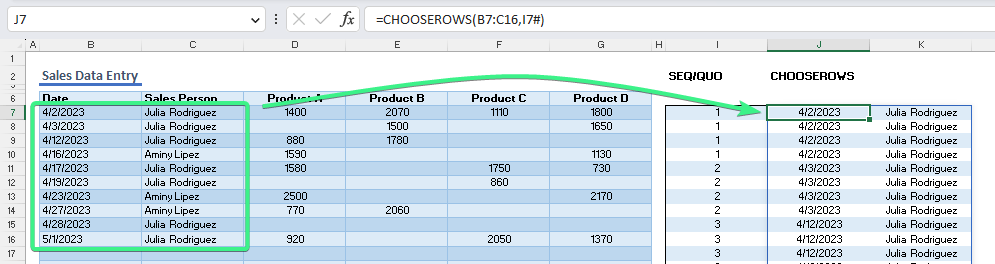Another way to multiply labels is a function named EXPAND. The EXPAND function expands an array to the specified row and column dimensions.

`=EXPAND(array, rows, [columns], [pad_with])`

In our example, we need to expand the headers for 10 rows. Subsequently, we can utilize the TOCOL function to distribute headers to rows. However, due to missing data, Excel populates empty rows with #N/A errors.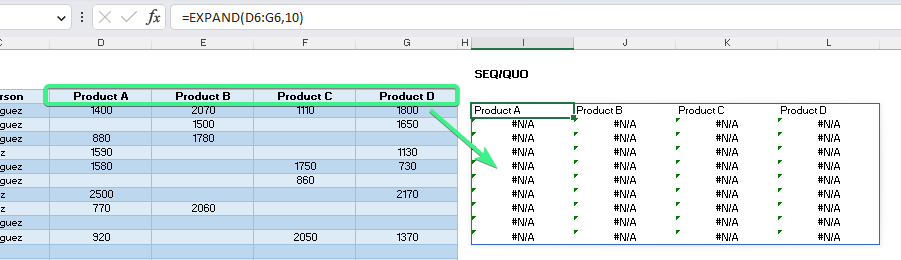Fortunately, with the aid of the IFNA function, we can conveniently substitute the errors with the headers. The IFNA function works by returning a specified value if the provided formula results in #N/A. You just need to include the header as the second argument too.

`=IFNA(EXPAND(D6:G6,10),D6:G6)`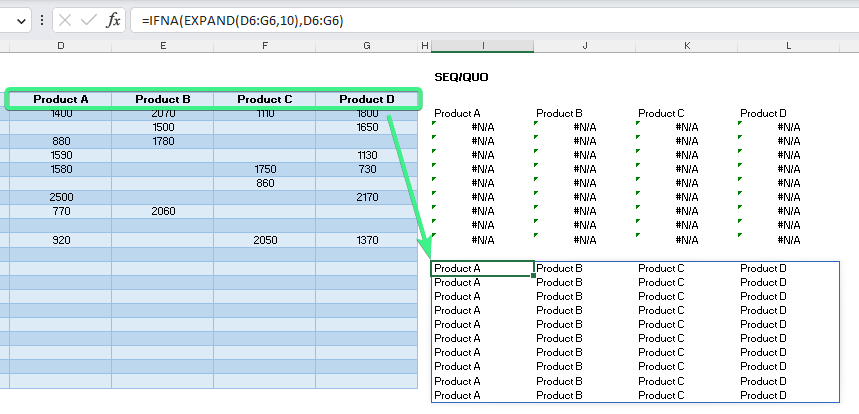At his point, we can utilize TOCOL to transform the array into a single column and merge the data. The image below demonstrates how the original data is unpivoted.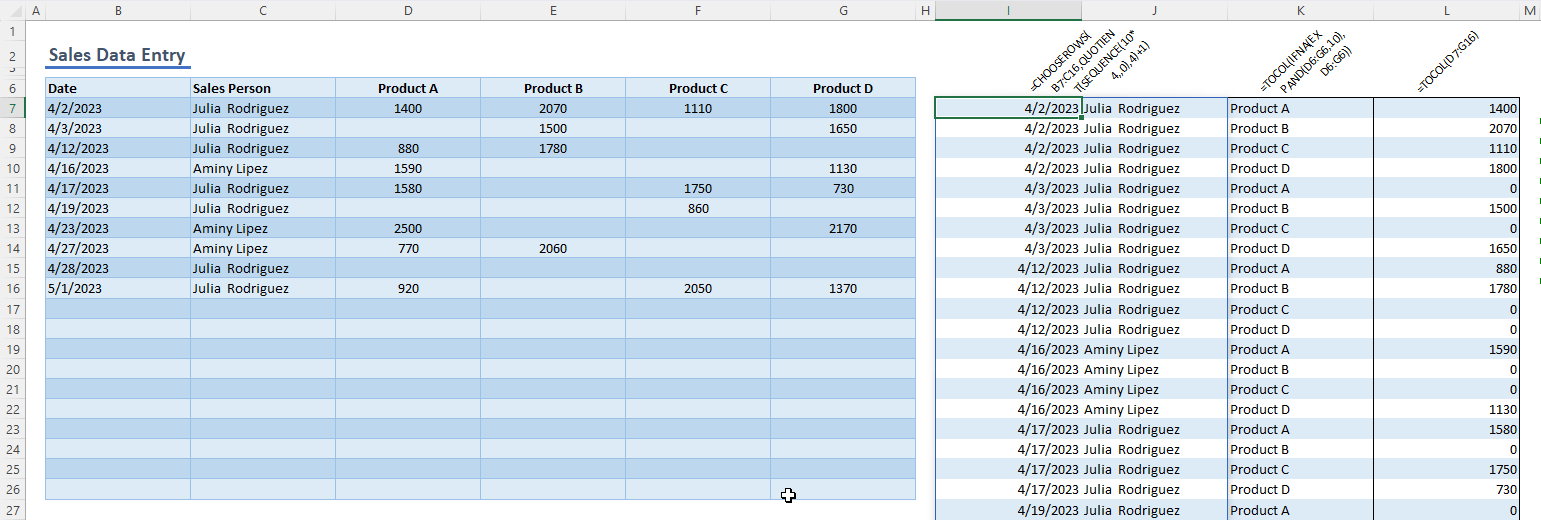While the outcome is as desired, relying on three separate formulas isn't an efficient way to unpivot. Alternatively, we can merge all these formulas into a single formula by using array combining functions such as VSTACK and HSTACK.

```=VSTACK(array1,[array2],...)
=HSTACK(array1,[array2],...)```

The VSTACK and HSTACK functions allow you to merge multiple arrays vertically and horizontally. For example, a raw combination of formulas will result in the same array:

`=HSTACK(CHOOSEROWS(B7:C16,QUOTIENT(SEQUENCE(10*4,,0),4)+1),TOCOL(IFNA(EXPAND(D6:G6,10),D6:G6)),TOCOL(D7:G16))`

VSTACK allows you to append headers to your table as well. All that's required is to supply a static array with the desired header ({"Date","Sales Person","Product","Sales Amount”}).

`=VSTACK({"Date","Sales Person","Product","Sales Amount"}, HSTACK(CHOOSEROWS(B7:C16,QUOTIENT(SEQUENCE(10*4,,0),4)+1),TOCOL(IFNA(EXPAND(D6:G6,10),D6:G6)),TOCOL(D7:G16)))`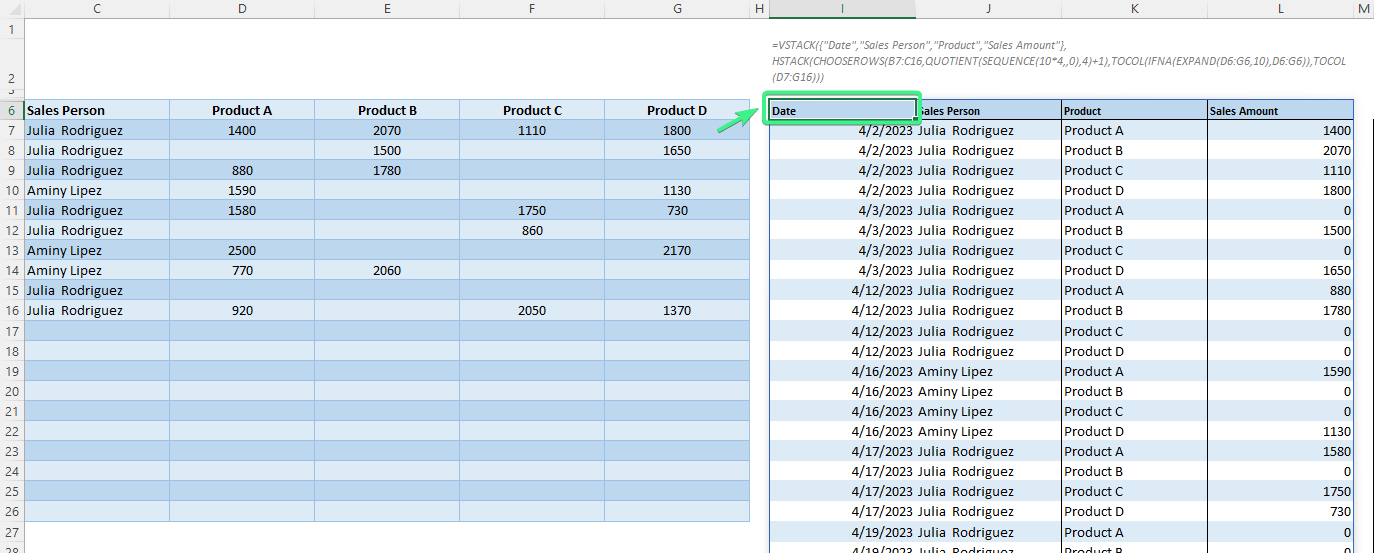You can even create a formula that only requires the table’s reference instead of separate parts of it like row labels, headers or values. Two new array manipulation functions, TAKE and DROP, allow you to extract a part of an array or omit (exclude) specific parts from the array.

```=TAKE(array, rows,[columns])
=DROP(array, rows,[columns])```

For example, in our case:

 Row Labels: TAKE(DROP(table,1),,2) Take first two columns of table without the first row. Column Labels (Headers): DROP(CHOOSEROWS(table,1),,2) Exclude first two columns from the first row. Values: DROP(table,1,2) Exclude first row and first two columns.
`=VSTACK({"Date","Sales Person","Product","Sales Amount"}, HSTACK( CHOOSEROWS(TAKE(DROP(table,1),,2),QUOTIENT(SEQUENCE(10*4,,0),4)+1), TOCOL(IFNA(EXPAND(DROP(CHOOSEROWS(B6:G16,1),,2),10),DROP(CHOOSEROWS(B6:G16,1),,2))), TOCOL(DROP(B6:G16,1,2))))`You can further boost the efficiency of the single-formula method by using the LET function’s in-formula named ranges. The LET function lets you define names within the scope of a formula. So, you can use names instead of references, preventing the need for repeated range selection.

`LET(name1, name_value1, calculation_or_name2, [name_value2, calculation_or_name3...])`

In our case we can refer to the table range (B6:G16) once.

```=LET(table,B6:G16,
VSTACK(
{"Date","Sales Person","Product","Sales Amount"},
HSTACK(
CHOOSEROWS(TAKE(DROP(table,1),,2),QUOTIENT(SEQUENCE(10*4,,0),4)+1),
TOCOL(IFNA(EXPAND(DROP(CHOOSEROWS(table,1),,2),10),DROP(CHOOSEROWS(table,1),,2))),
TOCOL(DROP(table,1,2)))))```For a fully automated experience, we can calculate and use the parameters for the helper functions such as SEQUENCE and QUOTIENT in the LET function.

The following formula only needs two inputs:

1. Table reference (B6:G26)
2. Number of columns that include row labels
```=LET(
raw_table,B6:G26,
label_count,2,
filled_table,FILTER(raw_table,NOT(ISBLANK(CHOOSECOLS(raw_table,1)))),
row_count,ROWS(filled_table)-1,
col_count,COLUMNS(filled_table)-label_count,
row_num,QUOTIENT(SEQUENCE(row_count*col_count,,0),col_count)+label_count,
VSTACK(
{"Date","Sales Person","Product","Sales Amount"},
HSTACK(
CHOOSEROWS(TAKE(filled_table,,label_count),row_num),
TOCOL(DROP(filled_table,1,label_count)))))```Lastly, we create a LAMBDA function that enables you to unpivot any data table of your choosing. Here is how you can test your LAMBDA function. Enter the formula and provide the argument within parentheses. The arguments consist of the reference and the count of the row labels:

```=LAMBDA(raw_table,label_count,
LET(
filled_table,FILTER(raw_table,NOT(ISBLANK(CHOOSECOLS(raw_table,1)))),
row_count,ROWS(filled_table)-1,
col_count,COLUMNS(filled_table)-label_count,
row_num,QUOTIENT(SEQUENCE(row_count*col_count,,0),col_count)+label_count,
VSTACK(
{"Date","Sales Person","Product","Sales Amount"},
HSTACK(
CHOOSEROWS(TAKE(filled_table,,label_count),row_num),`=Unpivot(B6:G26,2)`